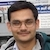# NAEST 2014 Screening Test: Newton's Laws and MagnetsBy

In NAEST 2014, there were two videos related to 2/3 magnets placed on a stand. The questions based on these videos give better insight into the Newton's laws of motion.

## Video 1

Question 1: Which of the pairs of magnets repel each other and which attract each other?

Question 2: What is the resultant force on magnet 2 and magnet 3?

Question 3: Why the separation between magnet 2 and 3 is more than the separation between magnet 1 and 2?

Answer: The free body diagram of three magnets is given in figure. The weight, $mg$, is same on all the three. The normal reaction from base on magnet 1 is $N$. Magnet 1 and 2 repel, also 2 and 3 repel, but 1 and 3 attract.Here, $F_{12}$ is magnetic force (repulsive) on magnet 1 due to magnet 2 and $F_{21}$ is magnetic force (repulsive) on magnet 2 due to magnet 1. By Newton's third law, $F_{12}=F_{21}$. Similarly, $F_{13}=F_{31}$ and $F_{23}=F_{32}$. Since magnets are stationary, Newton's second law gives resultant force on each magnet as zero. Thus, for magnet 1, \begin{align} F_{13}+N-F_{12}-mg=0, \end{align} for magnet 2, \begin{align} F_{21}-F_{23}-mg=0 \end{align} and for magnet 3, \begin{align} F_{32}-F_{31}-mg=0. \end{align} Substitute $F_{31}=F_{13}$, $F_{32}=F_{23}$ and $F_{21}=F_{12}$ to get \begin{align} &F_{13}+N-F_{12}-mg=0, \\ &F_{12}-F_{23}-mg=0, \\ &F_{23}-F_{13}-mg=0. \end{align} Solve to get, $N=3mg$ and $F_{12}=F_{23}+mg$. If stand is kept on a pan balance then pan balance will measure $N=3mg$. Also, repulsive force between 1 and 2 is greater than repulsive force between 2 and 3 ($F_{12}=F_{23}+mg$). This is possible when distance between 1 and 2 is less than distance between 2 and 3 (magnetic force increases as distance between magnets decreases).

## Video 2

Question 1: What is the mass of stand?

Question 2: What is the mass of magnet 1? Of magnet 2?

Question 3: What reading do you expect at the end?

Answer: The mass of the stand is $m=20$ g. Two magnets are identical with mass of each magnet $M=70$ g. Figure shows free body diagram of the stand and two magnets.The forces on the stand are its weight $mg$, reaction $N_1$ from magnet 1 and reaction $N_0$ from the pan balance. The forces on magnet 1 are its weight $Mg$, reaction from the stand $N_1^\prime$, and magnetic force on magnet 1 due to magnet 2, $F_{12}$. The forces on magnet 2 are its weight $Mg$ and magnetic force on magnet 2 due to magnet 1, $F_{21}$.

By Newton's third law, $N_{1}^\prime=N_1$ and $F_{21}=F_{12}$. Since each object is stationary, by Newton's second law, resultant force on each of them is zero. Thus, \begin{align} &N_0=N_1+mg, \\ &N_1^\prime=F_{12}+Mg \\ &F_{21}=Mg. \end{align} Substitute $F_{21}=F_{12}$ and $N_{1}^\prime=N_1$ and then solve to get \begin{align} N_0=mg+2Mg. \end{align} Again, by Newtons third law, reaction on the pan balance by the stand is \begin{align} N_0=mg+2Mg=(m+2M)g. \end{align} The mass measured by the pan balance is \begin{align} N_0/g=m+2M=20+2\times70=160\,\text{g}. \end{align}